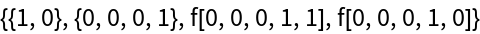#Function Repository Resource:

# TraversalOrderSelect

Choose positions of an expression by certain traversal-ordering criteria

Contributed by: Wolfram Research
 ResourceFunction["TraversalOrderSelect"][list,scheme] sorts elements from list according to a specified traversal scheme.

## Details and Options

The input list is typically a list of lists. This function was created to generate different evaluation schemes for combinators, and therefore is intended to sort positions at which updates could occur in a combinator expression.
In scheme, both direction ("Leftmost" or "Rightmost") and depth order ("Outermost" or "Innermost") must be specified, in order of their priority as sorting criteria.
The scheme, which is a list, can include an optional third element, specified as an integer number or Infinity, that indicates how many elements from the sorted list should be given.

## Examples

### Basic Examples (4)

Sort a list of positions in leftmost outermost order:

 In:=Out=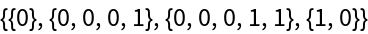Note that the order of criteria given in the scheme matters for sorting:

 In:=Out=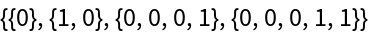Return only the first position from the list sorted by rightmost innermost order:

 In:=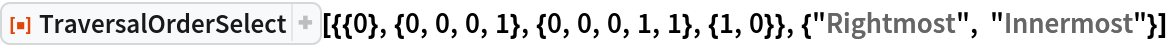Out=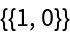Specify that two positions should be taken from the list sorted by innermost rightmost order:

 In:=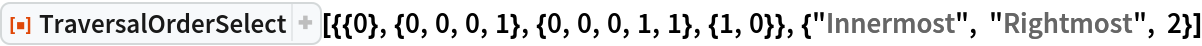Out=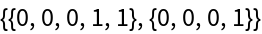### Scope (2)

If the third element of scheme is greater than the length of list, the fully sorted list is given:

 In:=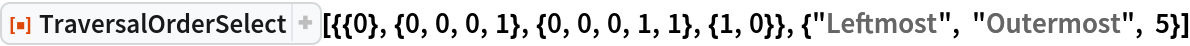Out=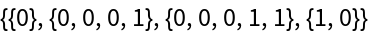Select the third position from the leftmost outermost sorting order:

 In:=Out=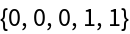### Possible Issues (1)

While this function was created to sort a list of lists, it can handle list elements with any arbitrary Head. In this example, the different heads are lexicographically ordered while the parts remain sorted by direction and depth order:

 In:=Out=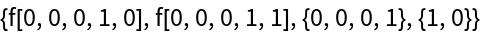In:=Out=